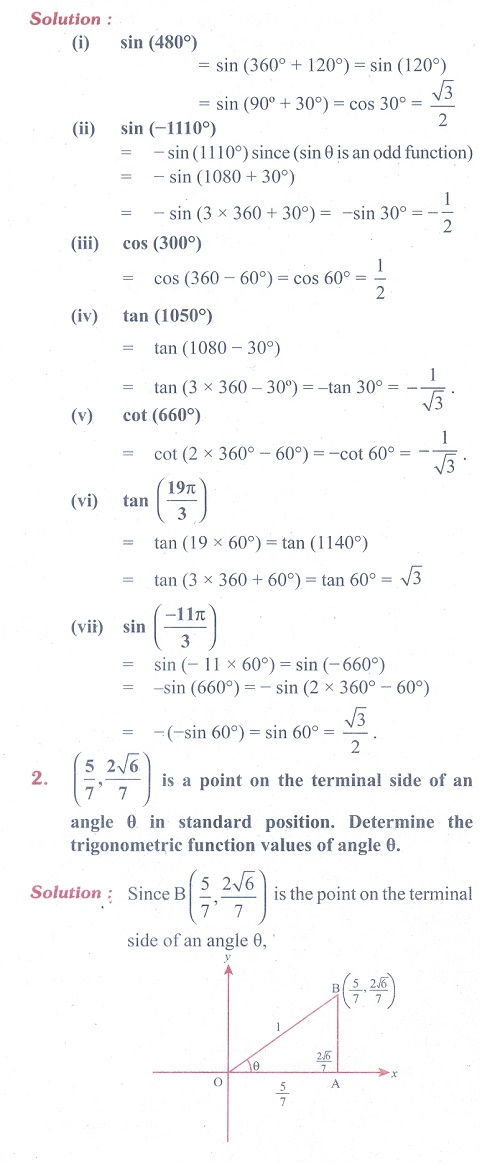Home | | Maths 11th std | Exercise 3.3: Trigonometric functions and their properties

# Exercise 3.3: Trigonometric functions and their properties

Maths Book back answers and solution for Exercise questions - Mathematics : Trigonometry: Trigonometric functions and their propertiesTags : Problem Questions with Answer, Solution | Mathematics , 11th Mathematics : UNIT 3 : Trigonometry
Study Material, Lecturing Notes, Assignment, Reference, Wiki description explanation, brief detail
11th Mathematics : UNIT 3 : Trigonometry : Exercise 3.3: Trigonometric functions and their properties | Problem Questions with Answer, Solution | Mathematics